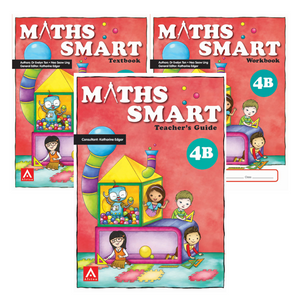or 6 weekly interest-free payments from \$30.83 withwhat's this?Alston Publishing House

# Maths Smart Level 4B

Regular price \$28.00 \$0.00 Unit price per
Tax included. Shipping calculated at checkout.

The Maths SMART series comprehensively covers the Learning outcomes in the Cambridge Primary Curriculum framework using the very popular teaching style of Singapore Maths.

Concepts and key skills within each Chapter are developed in a structured and step by step manner following the popular Concrete > Pictorial > Abstract approach to help pupils gain a firm foundation in mathematics. Well-crafted questions and activities are infused throughout the package to allow pupils to reinforce concepts learnt and enable them to acquire key skills such as creativity, critical thinking and problem-solving skills.

The System consists of 3 books working together to comprehensively teach each Maths concept: Teachers Guide, Student Textbook & Student Workbook

Teachers Guide: Provides an array of information, ideas and resources for complete teaching support. Every chapter includes :

1. Scheme of Work & Chapter Overview
2. Lesson Notes
3. Supportive Teachers guide features
Student Textbook: Packed with interesting features including
1. Chapter Opener
2. Learning Outcomes
3. "Do you Remember?" questions
4. Fascinating Facts
5. Use of concrete materials and pictorials
6. "Try This" Sections
7. Hands on Maths Ideas
9. Workbook links to correct workbook pages
10. "Apply what you know" sections
11. "I know that" key concept summary

Student Workbook:

1. Worksheets - exercises and activities
2. Putting it Together 1 - skill set questions
3. Putting it Together 2 - Problem solving, My thoughts & Self Check
4. Mid year review paper

Maths Smart 4B covers:

• Mental Skills: addition & subtraction, finding doubles & halves, multiplication
• Fractions: mixed numbers, improper fractions, converting fractions
• Decimals: understanding tenths & hundredths, comparing & ordering decimals, number patterns, rounding decimals, fractions & decimals
• Measurement: centimetres & millimetres, converting, measurement using decimals.
• Time: adding & subtracting, duration in hours & minutes.
• Area & Perimeter: finding area in square & standard units, finding perimeter, comparing areas & perimeters
• Handling Data: carroll & venn diagrams